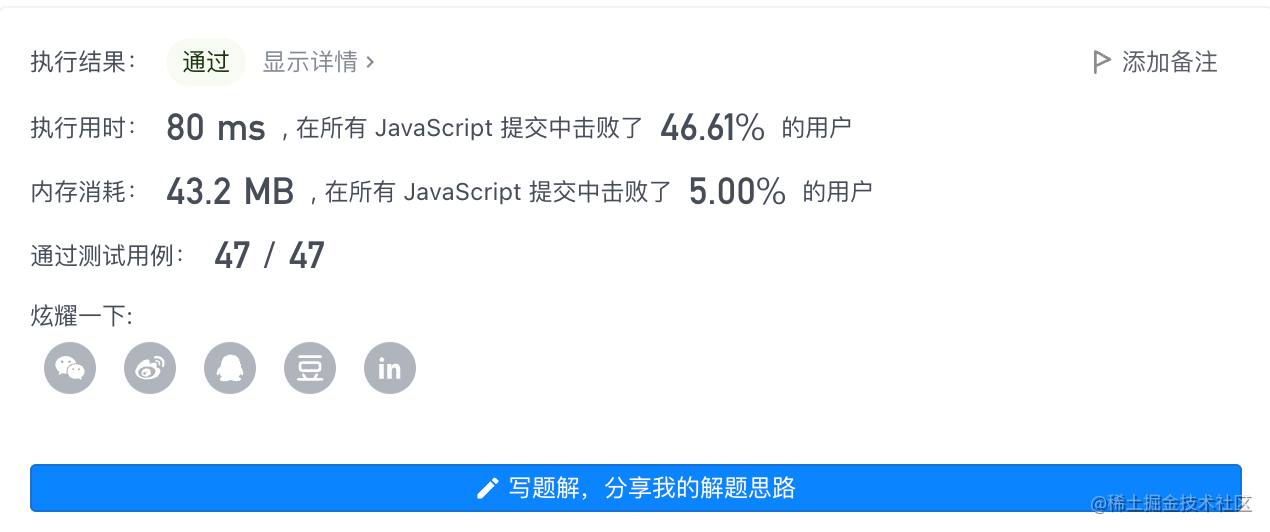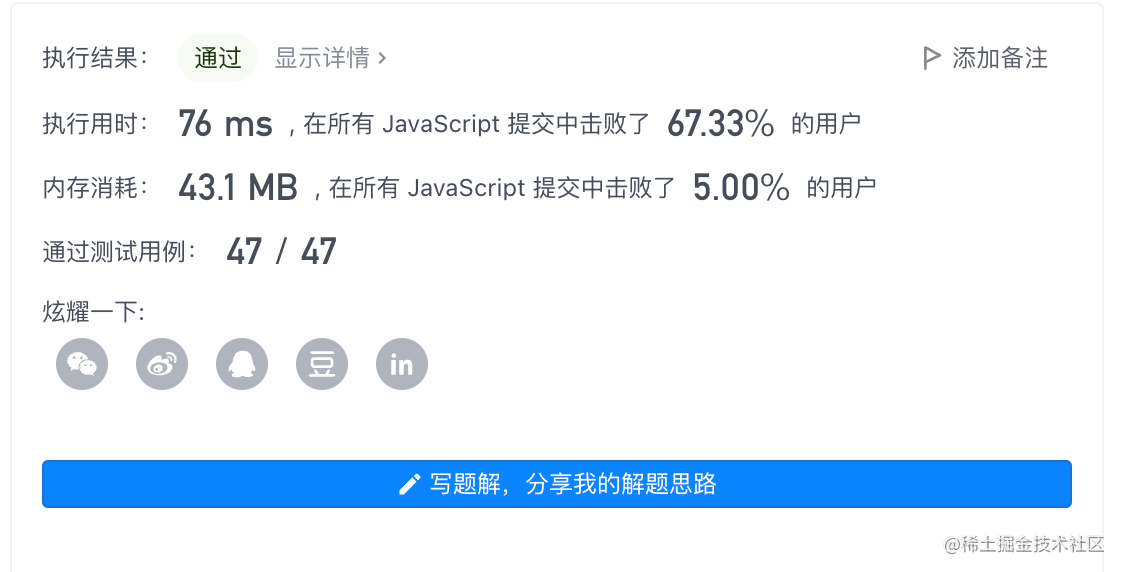+关注继续查看

# 1. 题目

输入： [3,2,3]

输入： [2,2,1,1,1,2,2]

# 2. 解一：哈希表

/**
* @param {number[]} nums
* @return {number}
*/
var majorityElement = function(nums) {
const a = []
const n = nums.length >> 1
for(let index in nums) {
a[nums[index]] = a[nums[index]] !== undefined ? ++a[nums[index]] : 1
if(a[nums[index]] > n) {
return nums[index]
}
}
};


• 时间复杂度: O(n)
• 空间复杂度: O(n)# 3. 投票算法Boyer-Moore

• 我们维护一个候选众数 candidate 和它出现的次数 count。初始时 candidate 可以为任意值，count 为 0
• 我们遍历数组 nums 中的所有元素，对于每个元素 nums，在判断 x 之前，如果 count 的值为 0，我们先将 x 的值赋予 candidate，随后我们判断 x

• 如果 xcandidate 相等，那么计数器 count 的值增加 1
• 如果 xcandidate 不等，那么计数器 count 的值减少 1
• 因为题目假设数组总是存在多数元素，所以在遍历完成后，candidate 即为整个数组的众数。
/**
* @param {number[]} nums
* @return {number}
*/
var majorityElement = function(nums) {
let count = 0, candidate
for(let i in nums) {
if(count === 0) {
candidate = nums[i]
}
count =  (nums[i] === candidate) ? ++count : --count
}
return candidate
};

• 时间复杂度： O(n)
• 空间复杂度： O(1)LeetCode 34. 在排序数组中查找元素的第一个和最后一个位置
LeetCode 34. 在排序数组中查找元素的第一个和最后一个位置
17 0LeetCode 169. 多数元素
LeetCode 169. 多数元素
15 0【LeetCode】 34. 在排序数组中查找元素的第一个和最后一个位置
34. 在排序数组中查找元素的第一个和最后一个位置
9 020 0leetcode 34 在排序数组中查找元素的第一个和最后一个位置
leetcode 34 在排序数组中查找元素的第一个和最后一个位置
8 0

JavaScript 自学手册文档教程323065

JavaScript入门与实战1921052

Javascript中的函数

Javascript异步编程

JS零基础入门教程（上册）